Published

# Web-based Garage Controller

Internet based garage controller with auto-close. Interact via web interface to see status, toggle, change auto-close time, and last action.

IntermediateWork in progress2 hours2,013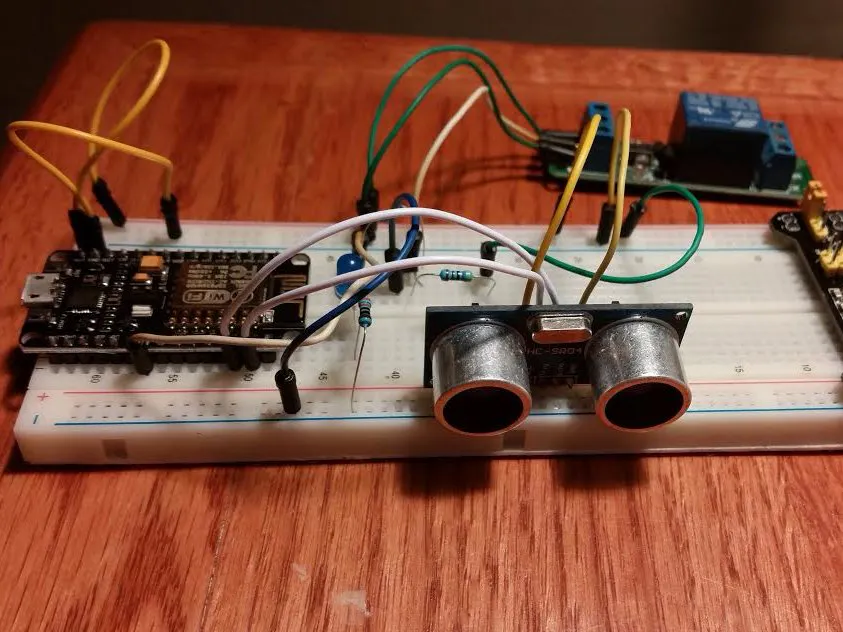## Things used in this project

### Hardware components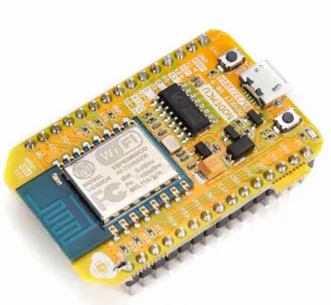NodeMCU ESP8266 Breakout Board
×1Ultrasonic Sensor - HC-SR04 (Generic)
×1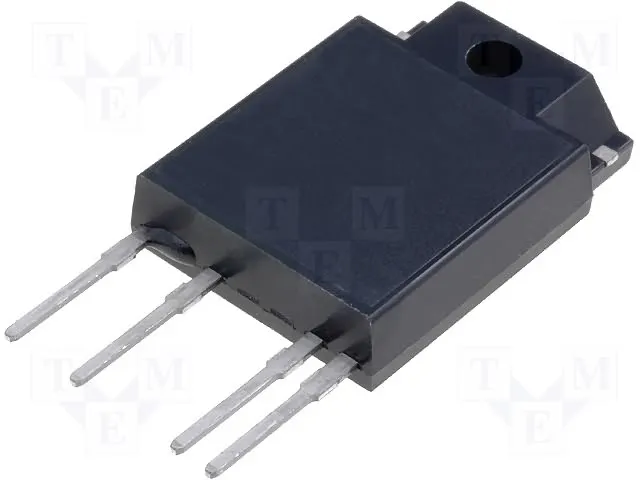Solid State Relay Any relay will do. For simplicity, using a high level trigger relay would be best due to the Arduino putting all the pins to LOW while being reset and other non-operational times.
×1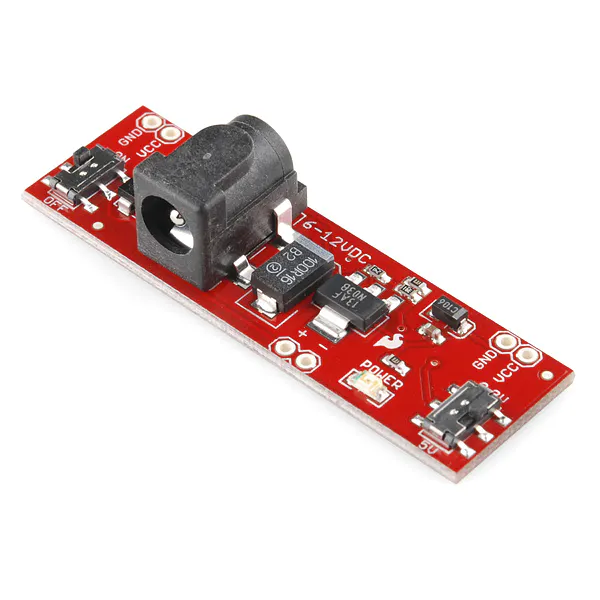SparkFun Breadboard Power Supply Stick 5V/3.3V
×1

## Schematics

### garagechecker_bb.png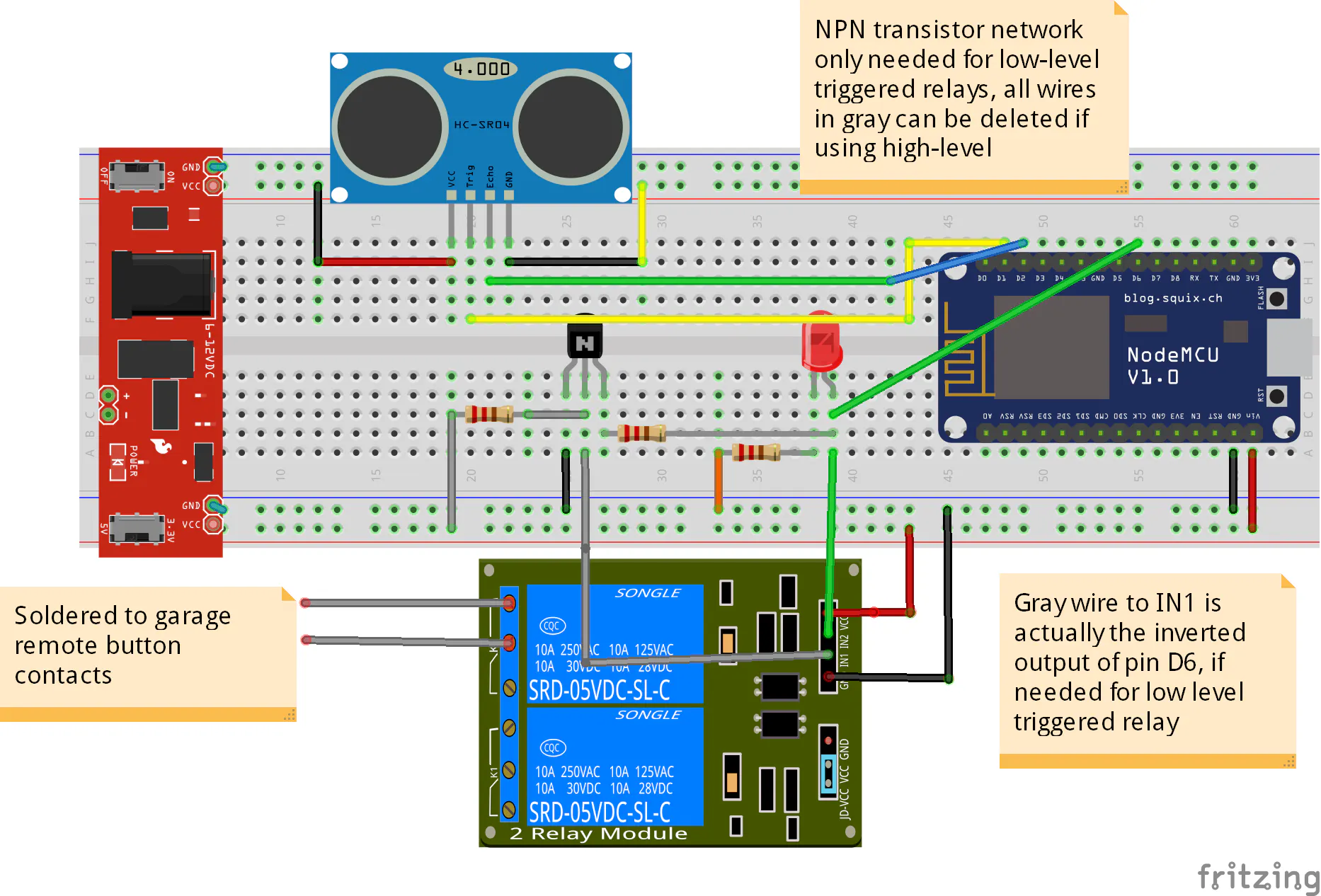## Code

### Web Based Garage Checker

Arduino
```/* Updated January 7, 2017
For closed is greater than threshold, vertical mount
This sketch uses a sonic sensor to detect a certain distance
like a garage door being open or closed
Features include:
Auto close after threshold(minutes) time
Distance threshold(in cm)
Error checking, 1 or 2 erroneous readings should not affect anything
Web interface to show if door is open or closed
Logs when door is opened or closed, and how it was opened or closed and grabs time from internet

Default values:
Auto-check every 10 seconds
If closed for over 6 readings (or one minute by default) then reset the counter

Error checking feature - if one reading is wrong, then it will not affect

Example-

Garage is closed
Check 1 (0 sec)  - Correct measurement of 23 cm    -Closed (1 out of 6 to reset)
Check 2 (10 sec) - Correct measurement of 23 cm    -Closed (2 out of 6)
Check 3 (20 sec) - Correct measurement of 23 cm    -Closed (3 out of 6)
Check 4 (30 sec) - Erroneous measurement of 1548cm -Open (2 out of 6)
Check 5 (40 sec) - Correct measurement of 23 cm    -Closed (3 out of 6)
Check 6 (50 sec) - Correct measurement of 23 cm    -Closed (4 out of 6)
Check 7 (60 sec) - Correct measurement of 23 cm    -Closed (5 out of 6)
Check 8 (70 sec) - Correct measurement of 23 cm    -Closed (6 out of 6) resets timer to 0
*/

#include <NTPClient.h>
#include <ESP8266WiFi.h>
#include <WiFiUdp.h>
#include <ESP8266mDNS.h>
#include <ArduinoOTA.h>

//const int testPin = 13;           //uysed to test door open or closed TEST ONLY

const int trigPin = 5;
const int echoPin = 4;
const int relay = 15;                 //garage remote relay pin
const int remotepin = 14;             //remote pin input
byte refresh = 0;                     //does the page need to be refreshed?
unsigned long timer = 0ul;            //timer updated every 10 to see if door has been open
int check;                            //used to hold average distance #
byte threshold = 40;                  //cm threshold for door open or close, if >= this then it is open
byte closedcount;                     //holds how many times should the door be counted closed before timer is reset
unsigned int minstoclose = 5u;       //default auto-close timer
byte autoclose = 0;                   //autoclose flag to display time since autoclose/manual toggle
unsigned long autoclosetimer = 0ul;   //how long since autoclose/manual toggle
unsigned long timeopen = 0ul;         //used to hold 30 seconds for threshold of garage door remote open
byte remoteopenthreshold = 30;        //seconds to disable remote opening
byte checkinterval = 10;              //interval in seconds for checking the door
byte autoclosethreshold = 6;          // how many checks to make sure door is closed
int  remotelock = 1;

boolean savings = 0;                  //daylight savings
int dataSize = 50;                    //size of array
String timestamp;                 //array of string to hold all timestamps
int pointer = 0;                      //array pointer
unsigned long prevUpdate;             //holds last update time for unix time update
boolean doorLastState;                //holds variable if door is open or closed

const char* ssid = "ssid";

WiFiUDP ntpUDP;
NTPClient timeClient(ntpUDP);

// Create an instance of the server
// specify the port to listen on as an argument
WiFiServer server(80);

void setup() {
//Serial.begin (115200);        //COMMENT OUT FOR TEST ONLY
delay(10);

pinMode(trigPin, OUTPUT);
pinMode(echoPin, INPUT);
pinMode(relay, OUTPUT);
pinMode(remotepin, INPUT);

// Connect to WiFi network
Serial.print("Connecting to ");
Serial.println(ssid);

WiFi.mode(WIFI_STA);

while (WiFi.waitForConnectResult() != WL_CONNECTED) {
Serial.println("Connection Failed! Rebooting...");
delay(5000);
ESP.restart();
}

ArduinoOTA.onStart([]() {
Serial.println("Start");
});
ArduinoOTA.onEnd([]() {
Serial.println("\nEnd");
});
ArduinoOTA.onProgress([](unsigned int progress, unsigned int total) {
Serial.printf("Progress: %u%%\r", (progress / (total / 100)));
});
ArduinoOTA.onError([](ota_error_t error) {
Serial.printf("Error[%u]: ", error);
if (error == OTA_AUTH_ERROR) Serial.println("Auth Failed");
else if (error == OTA_BEGIN_ERROR) Serial.println("Begin Failed");
else if (error == OTA_CONNECT_ERROR) Serial.println("Connect Failed");
else if (error == OTA_END_ERROR) Serial.println("End Failed");
});
ArduinoOTA.begin();
Serial.println("");
Serial.println("WiFi connected");

// Start the server
server.begin();
Serial.println("Server started");

Serial.println(WiFi.localIP());

timeClient.begin();
timeClient.update();
prevUpdate = millis();

check = distance();
if (check < threshold) {
doorLastState = 1;
} else {
doorLastState = 0;
}
}

void loop() {
ArduinoOTA.handle();
// Check if a client has connected

WiFiClient client = server.available();
if (!client) {
timer++;

if (digitalRead(remotepin) == HIGH && timer % 100 == 0 && remotelock == 0) {
if (millis() - timeopen > (remoteopenthreshold * 1000)) {
Serial.print("Pin is High and distance is");
check = distance();
Serial.println(check);
if (check < threshold) {
toggle(3);
Serial.println("Opening");
autoclose = 2;
autoclosetimer = 0;
timer = 0;
timeopen = millis();
}
} else {
Serial.println("Less than 30 seconds");
}
}

if (timer % (100 * checkinterval) == 0) { //this is every 10ms*this value, currently 10 seconds
if (autoclose == 1 || autoclose == 2) {
autoclosetimer++; //count up
}
Serial.print(timer / 100);
Serial.println(" seconds");

check = distance();   //every instance check for distance
if (check >= threshold) //runs if closed, closed count goes up
{
closedcount++;
Serial.print("Door is closed...");
Serial.println(closedcount);

if (closedcount >= autoclosethreshold) //if the count reaches 10 times then door is really closed
{ // and reset timer and closed counter
timer = 0;
closedcount = 0;
}

if (doorLastState == 1) { //was the door open?
delay(50);
check = distance();     //double check that is in fact closed
if (check >= threshold) {
doorLastState = 0;    //if so, show closed and log it
logData(4);
}
}

} else if (check < threshold) {
if (closedcount > 0) {
closedcount--; //decrease closedcount if door is found to be open but not less than 0
}

if (doorLastState == 0) {
delay(50);
check = distance();
if (check < threshold) {
doorLastState = 1;
logData(5);
}
}
}
}

if (timer >= 6000 * minstoclose && check < threshold) { //timer has reached it's threshold and the door is still open
Serial.println("reached threshold");
check = distance();
if (check < threshold) {
toggle(2);
Serial.println("Closing");
autoclose = 1;
autoclosetimer = 0;

}
timer = 0;
closedcount = 0;
}

if (millis() - prevUpdate >= 86400000 || timeClient.getEpochTime() < 1000000000ul) { //update time with NTP server every 24 hours
timeClient.update();
prevUpdate = millis();
}

delay(10);    //delay 10ms between every "check" to see if client has connected
return;       //also used as the minimum time unit for anything to happen
}

// Wait until the client sends some data
Serial.println("new client");
int timeout = 0;
while (!client.available()) {
delay(1);
timeout++;
if (timeout == 5000)
{
Serial.println("timeout");
client.flush();
delay(10);
return;
}
}

// Read the first line of the request
Serial.println(req);

if (req.indexOf("/?threshold=") != -1)
{
int pos = req.indexOf("threshold=");
Serial.println("Changing threshold");
minstoclose = req.substring(pos + 10, pos + 12).toInt();    //only looks at the 2 digits after the =
//refresh = 1;
if (minstoclose == 0) {     //just make it a long time if 0 or negative
minstoclose = 99999;      //this is around 70 days
}
//timestamp[pointer] =  getTime() + " Auto close threshold changed: " + String(minstoclose) + " minutes";
logData(6);
}

if (req.indexOf("/?command=Toggle") != -1)  //if it sees ?command=Toggle
{
toggle(1);
autoclose = 2;                            //toggle flag
autoclosetimer = 0;                       //reset the timer for toggle
timer = 0;                                //reset the threshold timer
refresh = 1;
}

if (req.indexOf("/?remotelock=") != -1)
{
int pos = req.indexOf("remotelock=");
Serial.print("Remote lock ");
remotelock = req.substring(pos + 11, pos + 12).toInt();    //only looks at the 1 digits after the =
refresh = 1;
}

if (req.indexOf("/?command=Clear") != -1)
{
logData(0);
refresh = 1;
}

String s = webpage();

// Send the response to the client
client.print(s);
delay(10);

client.flush();
// The client will actually be disconnected
// when the function returns and 'client' object is destroyed
}

String webpage() {
String s = "HTTP/1.1 200 OK\r\nContent-Type: text/html\r\n\r\n<!DOCTYPE HTML>\r\n<html>\r\n<head><title>Remote Garage Door</title>\r\n";

if (refresh == 1) {
//s += "<meta charset=\"UTF-8\">\r\n\<script type=\"text/javascript\">\r\nhistory.back();\r\n</script>\r\n";
s += "<script type=\"text/javascript\">location.href = \"../\"</script>\r\n";
refresh = 0;
}

s += "</head>\r\nGarage Door Status: ";
int check = distance();
if (check < threshold) {
s += "OPEN";
} else {
s += "CLOSED";
}

s += "\r\n<br><br><form type=\"get\" name=\"toggle\">\r\n<input type=\"submit\" name=\"command\" value=\"Toggle\">\r\n</form>\r\n";

s += "<br><br>\r\n";
if (autoclose == 1)  //if it autoclosed last-display the minutes since auto closed
{
//s += String(autoclosetimer * checkinterval / 60);
s += String((millis() - timeopen) / (60 * 1000));
s += " minutes since last Auto Close\r\n<br><br><br>\r\n";
} else if (autoclose == 2) {  //if it was toggled, display minutes since last toggled
//s += String(autoclosetimer * checkinterval / 60);
s += String((millis() - timeopen) / (60 * 1000));
s += " minutes since last Toggle\r\n<br><br>\r\n";
}

s += "<form type=\"get\" action\"/\"><input type=\"text\" name=\"threshold\" value=\"";
s += minstoclose;
s += "\"><input type=\"submit\" value=\"Change Auto-Close Time\"></form>\r\n";
s += "<br><form type=\"get\" action=\"/\"><input type=\"submit\" value=\"Refresh\">\r\n</form><br><br>\r\n";

s += String(check);
s += " cm\r\n<br><br>\r\n";
s += String(timer / 100);
s += " seconds\r\n\<br><br>\r\n";
s += String(minstoclose);
s += " minute threshold or ";
s += String(minstoclose * 60);
s += " seconds<br>\r\n\<br>\r\n";
if (!remotelock) {
s += "<br>Remote Auto Open ON<br>\r\n";
}

for (int asdf = pointer + dataSize - 1; asdf >= pointer; asdf--)
{
if (timestamp[asdf % dataSize] != "")
{
s += timestamp[asdf % dataSize];
s += "<br>\r\n";
}
}

s += "<br>\r\n<form type=\"get\" name=\"clear\">\r\n<input type=\"submit\" name=\"command\" value=\"Clear Log\">\r\n</form>\r\n<br>\r\n";

if (check < 125 && check > 90) {
s += "Nicole's car is in<br>\r\n";
} else if (check > 130 && check < 145) {
s += "Eric's car is in<br>\r\n";
}

s += "</html>\n";
return s;
}

int distance() {
long duration, distance;
digitalWrite(trigPin, LOW);
delayMicroseconds(2);
digitalWrite(trigPin, HIGH);
delayMicroseconds(10);
digitalWrite(trigPin, LOW);
duration = pulseIn(echoPin, HIGH);
distance = (duration / 2) / 29.1;

Serial.print(distance);
Serial.println(" cm");
/*
long distance;                              //TEST ONLY
if (digitalRead(testPin) == LOW) {          //used for test bench
distance = 39;
} else {
distance = 41;
}
*/
if (distance == 0) {
return (threshold + 2);
}
return distance;

}

void toggle(int tog) {
Serial.print("Toggle");
digitalWrite(relay, HIGH);        //toggle the garage door
delay(500);                       //hold it for half a second to make sure it gets a good "press"
digitalWrite(relay, LOW);
Serial.println("d");
timeopen = millis();

logData(tog);
return;
}

String getTime() {
unsigned long rawtime = timeClient.getEpochTime();

if (rawtime < 1000000000ul) {
return "Clock Error: ";
}

rawtime = rawtime - (18000ul + (!savings * 3600ul));

unsigned long day = ((rawtime / 86400L) + 4) % 7;
String dayStr;
switch (day) {
case 0:
dayStr = "Sunday";
break;

case 1:
dayStr = "Monday";
break;

case 2:
dayStr = "Tuesday";
break;

case 3:
dayStr = "Wednesday";
break;

case 4:
dayStr = "Thursday";
break;

case 5:
dayStr = "Friday";
break;

case 6:
dayStr = "Saturday";
break;

default:
break;
}

unsigned long hours = (rawtime % 86400L) / 3600;
String hoursStr = hours < 10 ? "0" + String(hours) : String(hours);

unsigned long minutes = (rawtime % 3600) / 60;
String minuteStr = minutes < 10 ? "0" + String(minutes) : String(minutes);

unsigned long seconds = rawtime % 60;
String secondStr = seconds < 10 ? "0" + String(seconds) : String(seconds);

return dayStr + " " + hoursStr + ":" + minuteStr + ":" + secondStr;
}

void logData(int flag) {
if (flag == 1) {
timestamp[pointer] = getTime() + " Program Toggled";
} else if (flag == 2) {
timestamp[pointer] = getTime() + " Auto Closed";
} else if (flag == 3) {
timestamp[pointer] = getTime() + " Remote Toggle";
} else if (flag == 4) {
if (millis() - timeopen < 15000) {
timestamp[pointer - 1] += " - Door CLOSED";
pointer--;
} else {
timestamp[pointer] = getTime() + " Manual Toggle - Door CLOSED";
}
} else if (flag == 5) {
if (millis() - timeopen < 15000) {
timestamp[pointer - 1] += " - Door OPENED";
pointer--;
} else {
timestamp[pointer] = getTime() + " Manual Toggle - Door OPENED";
}
} else if (flag == 6) {
timestamp[pointer] = getTime() + " Auto Close Timer Changed: " + String(minstoclose) + " minutes";
}

pointer++;

if (pointer >= dataSize) {
pointer = 0;
}

if (flag == 0) {
for (int asdf = 0; asdf < dataSize; asdf++)
{
timestamp[asdf] = "";
}
pointer = 0;
}
return;
}
```